### Day 11 - Unit 1 Overview - 08.31.15

Update
• Unit 1 Test this Thursday!

Bell Ringer
1. Find the limit for the following: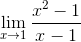1. undefined

2. 1

3. 2

4. 0

5. none of the above

2.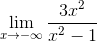1.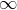2. 3

3. 0

4.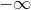5. none of the above

3. Find the following limit: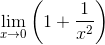1.2.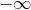3. 0

4. 1

5. none of the above

4. Find the x-values (if any) at which the function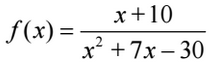is not continuous. Which of the discontinuities are removable?

1. no points of discontinuity.

2. x = –10 (not removable), x = 3 (removable)

3. x = –10 (removable), x = 3 (not removable)

4. no points of continuity.

5. x = –10 (not removable), x = 3 (not removable)

5. Find the limit (if it exists).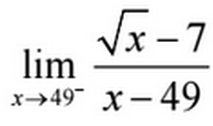1. 1 / 14

2. 0

3. 1 / 98

4. -1 / 14

5. limits does not exist

Review
• Math Overview (video)
• Numbers
• Relationships
• Shapes
• Change
• Limits
• Intro to Limits (video)
• Nonexistent Limits (video)
• How are limits found numerically and graphically? (checkpoints)
• How are limits found algebraically? (checkpoints)
• Continuity (videoand One-sided Limits (video)
• How can discontinuity of a function be described?
• How are one-sided limits related to regular limits? (checkpoints)
• Infinite Limits (video)
• Limits at Infinity (video)

Lesson
• Book Review
• Complete problems in the following sections
• 1.2 - Finding Limits Graphically and Numerically
• 1.3 - Evaluating Limits Analytically
• 1.4 - Continuity and One-sided Limits
• 1.5 - Infinite Limits

Exit Ticket
• Posted on the board at the end of the block

Homework
• N/A

Lesson Objectives
• Unit 1 Overview

#### In-Class Help Requests

Standard(s)
• APC.2
• Define and apply the properties of limits of functions.
• Limits will be evaluated graphically and algebraically.
• Includes:
• ​limits of a constant
• ​limits of a sum, product, and quotient
• ​one-sided limits
• ​limits at infinity, infinite limits, and non-existent limits*
• APC.3
• Use limits to define continuity and determine where a function is continuous or discontinuous.
• Includes:
• ​continuity in terms of limits
• continuity at a point and over a closed interval
• ​application of the Intermediate Value Theorem and the Extreme Value Theorem
• ​geometric understanding and interpretation of continuity and discontinuity